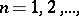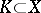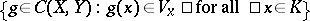# Pointwise convergence

(diff) ← Older revision | Latest revision (diff) | Newer revision → (diff)
A type of convergence of sequences of functions (mappings). Let,whereis some set andis a topological space; then pointwise convergence means that for any elementthe sequence of points,converges in the space. An important subclass of the pointwise-convergent sequences for the case of mappings between metric spaces (or, more generally, uniform spaces) is that of the uniformly-convergent sequences (cf. Uniform convergence).
A base for the topology of pointwise convergence on, the space of continuous mappings fromto, is obtained as follows. Take a finite setand for eachan open subset incontaining; for a givenan open basis neighbourhood is:. See also Pointwise convergence, topology of.